You are using an outdated browser. Please upgrade your browser to improve your experience.The Gaussian Probability Calculator utilizing the Gaussian Probability Function returns the probability that an entered random variable (x) has a probability value less than the entered (x) value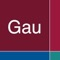Gaussian Probability

by Donald Schaefer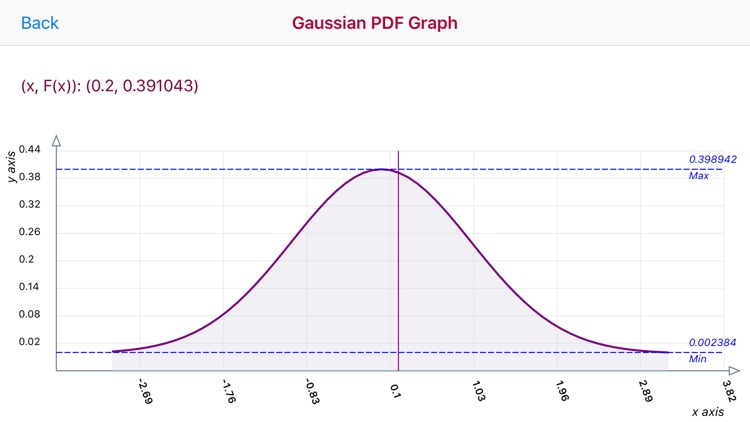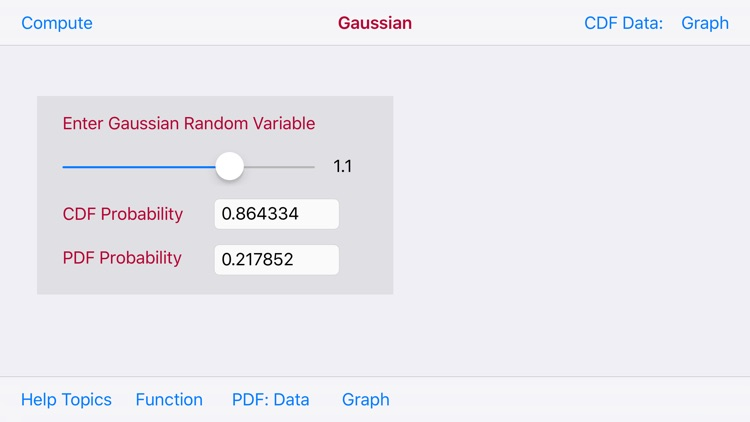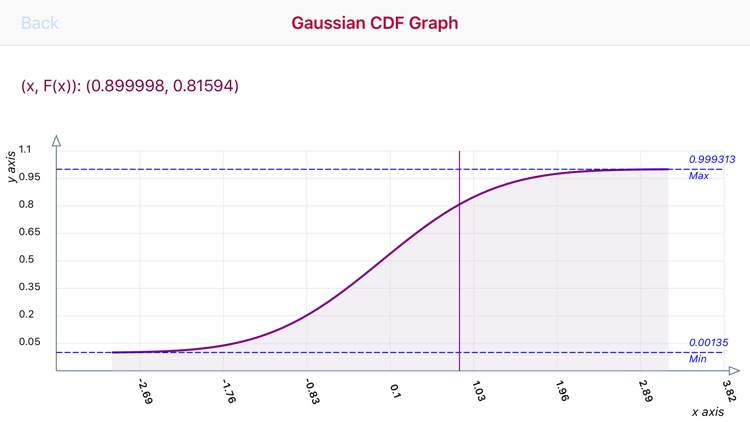Gaussian Probability Offers Apple Watch App
Check the details on WatchAware

What is it about?

The Gaussian Probability Calculator utilizing the Gaussian Probability Function returns the probability that an entered random variable (x) has a probability value less than the entered (x) value..App Details

Version
1.2
Rating
NA
Size
7Mb
Genre
Education Productivity
Last updated
March 2, 2019
Release date
February 10, 2016

App Screenshots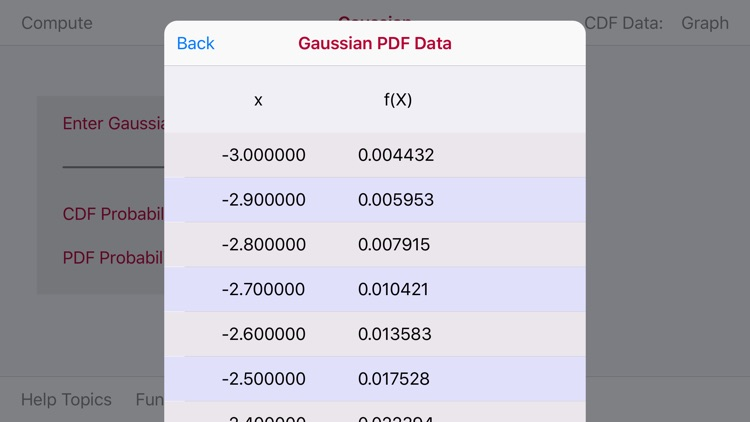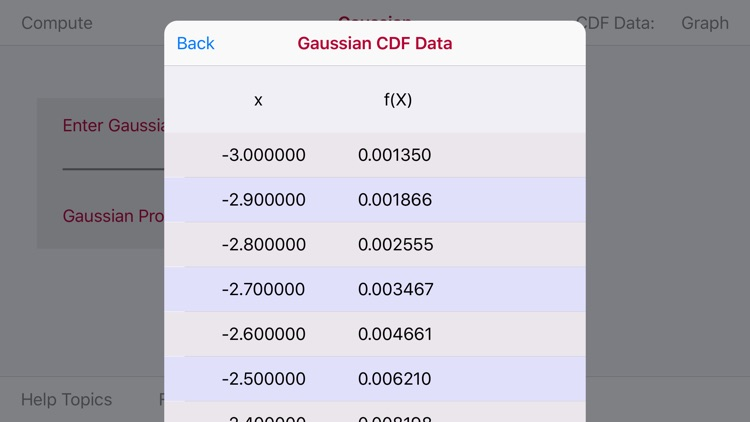App Store Description

The Gaussian Probability Calculator utilizing the Gaussian Probability Function returns the probability that an entered random variable (x) has a probability value less than the entered (x) value..

The random variable (x) is entered via a Slider with a data range of -3.1 to 3.0.

In addition the Gaussian Probability Calculator produces a PDF/CDF Graph and PDF/CDF Data Tables producing random variable (x) and the Gaussian probability f(x) a range of random variable (x) values of -3.00 to 3.20

The PDF and CDF graph are touch interactive graphs for computed (x/Pr(x) paired values. The graphs hav a touch feature whereby upon the touch a slidable vertical line appears. Upon movement of the line a paired (x,Pr(x) values appear relative to the line position on the graph curve.

The horizontal x-axis displays computed (x) values. The vertical y-axis plots a range of Pr(x) values.

Horizontal Max and Min dashed lines display the Maximum and Minimum F(x) values

The iOS 12 version add the ability to enter the (x) value on a numeric basis

Disclaimer:
AppAdvice does not own this application and only provides images and links contained in the iTunes Search API, to help our users find the best apps to download. If you are the developer of this app and would like your information removed, please send a request to [email protected] and your information will be removed.